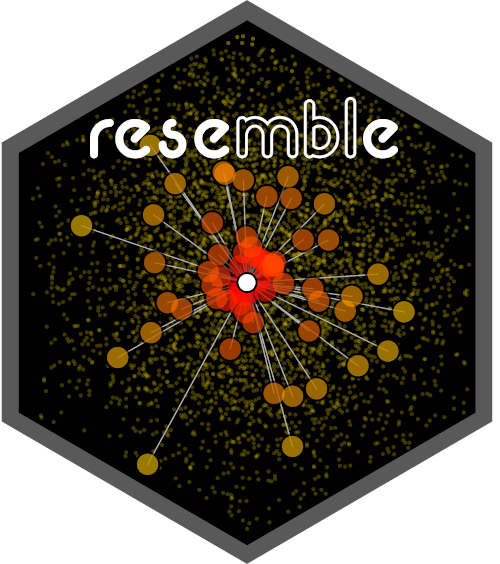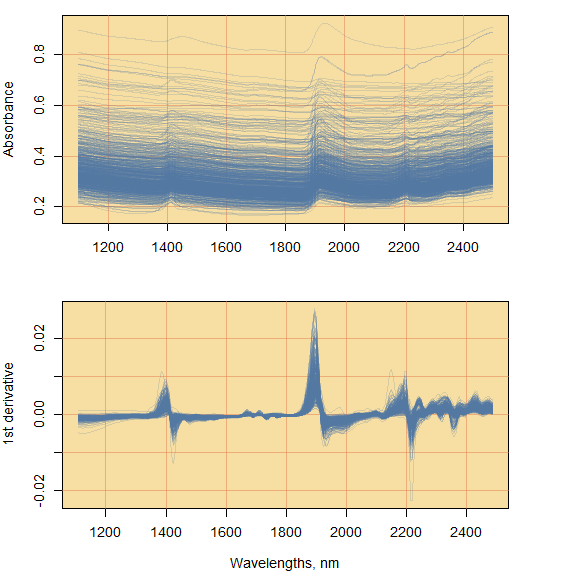Think Globally, Fit Locally (Saul and Roweis 2003)# 1 Introduction

Modeling spectral data has garnered wide interest in the last four decades. Spectroscopy is the study of the spectral response of a matrix (e.g. soil, plant material, seeds, etc.) when it interacts with electromagnetic radiation. This spectral response directly or indirectly relates to a wide range of compositional characteristics (chemical, physical or biological) of the matrix. Therefore, it is possible to develop empirical models that can accurately quantify properties of different matrices. In this respect, quantitative spectroscopy techniques are usually fast, non-destructive and cost-efficient in comparison to conventional laboratory methods used in the analyses of such matrices. This has resulted in the development of comprehensive spectral databases for several agricultural products comprising large amounts of observations. The size of such databases increases de facto their complexity. To analyze large and complex spectral data, one must then resort to numerical and statistical tools and methods such as dimensionality reduction, and local spectroscopic modeling based on spectral dissimilarity concepts.

The aim of the `resemble` package is to provide tools to efficiently and accurately extract meaningful quantitative information from large and complex spectral databases. The core functionalities of the package include:

• dimensionality reduction
• computation of dissimilarity measures
• evaluation of dissimilarity matrices
• spectral neighbour search
• fitting and predicting local spectroscopic models

# 2 Citing the package

Simply type and you will get the info you need:

``````citation(package = "resemble")
##
## To cite resemble in publications use:
##
##   Ramirez-Lopez, L., and Stevens, A., and Viscarra Rossel, R., and
##   Lobsey, C., and Wadoux, A., and Breure, T. (2020). resemble:
##   Regression and similarity evaluation for memory-based learning in
##   spectral chemometrics. R package Vignette R package version 2.0.0.
##
## A BibTeX entry for LaTeX users is
##
##   @Manual{,
##     title = {resemble: Regression and similarity evaluation for memory-based learning in spectral chemometrics. },
##     author = {Leonardo Ramirez-Lopez and Antoine Stevens and Raphael Viscarra Rossel and Craig Lobsey and Alex Wadoux and Timo Breure},
##     publication = {R package Vignette},
##     year = {2020},
##     note = {R package version 2.0.0},
##     url = {https://CRAN.R-project.org/package=resemble},
##   }``````

# 3 Example dataset

This vignette uses the soil Near-Infrared (NIR) spectral dataset provided in the package `prospectr` package (Stevens and Ramirez-Lopez 2020). The reason why we use this dataset is because soils are one of the most complex matrices analyzed with NIR spectroscopy. This spectral dataset/library was used in the challenge by Pierna and Dardenne (2008). The library contains NIR absorbance spectra of dried and sieved 825 soil observations/samples. These samples originate from agricultural fields collected from all over the Walloon region in Belgium. The data are in an `R` `data.frame` object which is organized as follows:

• Response variables:

• Nt (Total Nitrogen in g/kg of dry soil): a numerical variable (values are available for 645 samples and missing for 180 samples).

• Ciso (Carbon in g/100 g of dry soil): a numerical variable (values are available for 732 and missing for 93 samples).

• CEC (Cation Exchange Capacity in meq/100 g of dry soil): A numerical variable (values are available for 447 and missing for 378 samples).

• Predictor variables: the predictor variables are in a matrix embedded in the data frame, which can be accessed via `NIRsoil\$spc`. These variables contain the NIR absorbance spectra of the samples recorded between the 1100 nm and 2498 nm of the electromagnetic spectrum at 2 nm interval. Each column name in the matrix of spectra represents a specific wavelength (in nm).

• Set: a binary variable that indicates whether the samples belong to the training subset (represented by 1, 618 samples) or to the test subset (represented by 0, 207 samples).

Load the necessary packages and data:

``````library(resemble)
library(prospectr)
library(magrittr)``````

The dataset can be loaded into R as follows:

``````data(NIRsoil)
dim(NIRsoil)
str(NIRsoil)``````

# 4 Spectra pre-processing

This step aims at improving the signal quality of the spectra for quantitative analysis. In this respect, the following standard methods are applied using the package `prospectr` (Stevens and Ramirez-Lopez 2020):

1. Resampling from a resolution of 2 nm to a resolution of 5 nm.
2. First derivative using Savitsky-Golay filtering (Savitzky and Golay 1964).
``````# obtain a numeric vector of the wavelengths at which spectra is recorded
wavs <- NIRsoil\$spc %>% colnames() %>% as.numeric()

# pre-process the spectra:
# - resample it to a resolution of 6 nm
# - use first order derivative
new_res <- 5
poly_order <- 1
window <- 5
diff_order <- 1

NIRsoil\$spc_p <- NIRsoil\$spc %>%
resample(wav = wavs, new.wav = seq(min(wavs), max(wavs), by = new_res)) %>%
savitzkyGolay(p = poly_order, w = window, m = diff_order)``````Figure 4.1: Raw spectral absorbance data (top) and first derivative of the absorbance spectra (bottom).

``````new_wavs <- as.matrix(as.numeric(colnames(NIRsoil\$spc_p)))

matplot(x = wavs, y = t(NIRsoil\$spc),
xlab = "Wavelengths, nm",
ylab = "Absorbance",
type = "l", lty = 1, col = "#5177A133")

matplot(x = new_wavs, y = t(NIRsoil\$spc_p),
xlab = "Wavelengths, nm",
ylab = "1st derivative",
type = "l", lty = 1, col = "#5177A133")``````

Both the raw absorbance spectra and the first derivative spectra are shown in Figure 4.1. The first derivative spectra represents the explanatory variables that will be used for all the examples throughout this document.

For more explicit examples, the `NIRsoil` data is split into training and testing subsets: